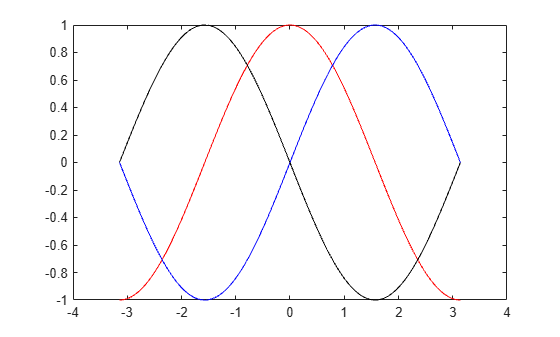# diff

Differences and approximate derivatives

## Syntax

``Y = diff(X)``
``Y = diff(X,n)``
``Y = diff(X,n,dim)``

## Description

example

````Y = diff(X)` calculates differences between adjacent elements of `X` along the first array dimension whose size does not equal 1:If `X` is a vector of length `m`, then `Y = diff(X)` returns a vector of length `m-1`. The elements of `Y` are the differences between adjacent elements of `X`.Y = [X(2)-X(1) X(3)-X(2) ... X(m)-X(m-1)]If `X` is a nonempty, nonvector p-by-m matrix, then `Y = diff(X)` returns a matrix of size (p-1)-by-m, whose elements are the differences between the rows of `X`.Y = [X(2,:)-X(1,:); X(3,:)-X(2,:); ... X(p,:)-X(p-1,:)]If `X` is a 0-by-0 empty matrix, then `Y = diff(X)` returns a 0-by-0 empty matrix.```

example

````Y = diff(X,n)` calculates the nth difference by applying the `diff(X)` operator recursively `n` times. In practice, this means `diff(X,2)` is the same as `diff(diff(X))`.```

example

````Y = diff(X,n,dim)` is the nth difference calculated along the dimension specified by `dim`. The `dim` input is a positive integer scalar.```

## Examples

collapse all

Create a vector, then compute the differences between the elements.

```X = [1 1 2 3 5 8 13 21]; Y = diff(X)```
```Y = 1×7 0 1 1 2 3 5 8 ```

Note that `Y` has one fewer element than `X`.

Create a 3-by-3 matrix, then compute the first difference between the rows.

```X = [1 1 1; 5 5 5; 25 25 25]; Y = diff(X)```
```Y = 2×3 4 4 4 20 20 20 ```

`Y` is a 2-by-3 matrix.

Create a vector and compute the second-order difference between the elements.

```X = [0 5 15 30 50 75 105]; Y = diff(X,2)```
```Y = 1×5 5 5 5 5 5 ```

Create a 3-by-3 matrix, then compute the first-order difference between the columns.

```X = [1 3 5;7 11 13;17 19 23]; Y = diff(X,1,2)```
```Y = 3×2 2 2 4 2 2 4 ```

`Y` is a 3-by-2 matrix.

Use the `diff` function to approximate partial derivatives with the syntax `Y = diff(f)/h`, where `f` is a vector of function values evaluated over some domain, `X`, and `h` is an appropriate step size.

For example, the first derivative of `sin(x)` with respect to `x` is `cos(x)`, and the second derivative with respect to `x` is `-sin(x)`. You can use `diff` to approximate these derivatives.

```h = 0.001; % step size X = -pi:h:pi; % domain f = sin(X); % range Y = diff(f)/h; % first derivative Z = diff(Y)/h; % second derivative plot(X(:,1:length(Y)),Y,'r',X,f,'b', X(:,1:length(Z)),Z,'k')```In this plot the blue line corresponds to the original function, `sin`. The red line corresponds to the calculated first derivative, `cos`, and the black line corresponds to the calculated second derivative, `-sin`.

Create a sequence of equally-spaced datetime values, and find the time differences between them.

```t1 = datetime('now'); t2 = t1 + minutes(5); t = t1:minutes(1.5):t2```
```t = 1x4 datetime Columns 1 through 3 31-Aug-2022 03:36:56 31-Aug-2022 03:38:26 31-Aug-2022 03:39:56 Column 4 31-Aug-2022 03:41:26 ```
`dt = diff(t)`
```dt = 1x3 duration 00:01:30 00:01:30 00:01:30 ```

`diff` returns a `duration` array.

## Input Arguments

collapse all

Input array, specified as a vector, matrix, or multidimensional array. `X` can be a numeric array, logical array, datetime array, or duration array.

Complex Number Support: Yes

Difference order, specified as a positive integer scalar or `[]`. The default value of `n` is 1.

It is possible to specify `n` sufficiently large so that `dim` reduces to a single (```size(X,dim) = 1```) dimension. When this happens, `diff` continues calculating along the next array dimension whose size does not equal 1. This process continues until a 0-by-0 empty matrix is returned.

Data Types: `single` | `double` | `int8` | `int16` | `int32` | `int64` | `uint8` | `uint16` | `uint32` | `uint64`

Dimension to operate along, specified as a positive integer scalar. If you do not specify the dimension, then the default is the first array dimension of size greater than 1.

Consider a two-dimensional p-by-m input array, `A`:

• `diff(A,1,1)` works on successive elements in the columns of `A` and returns a (p-1)-by-m difference matrix.

• `diff(A,1,2)` works on successive elements in the rows of `A` and returns a p-by-(m-1) difference matrix.Data Types: `double` | `single` | `int8` | `int16` | `int32` | `int64` | `uint8` | `uint16` | `uint32` | `uint64`

## Output Arguments

collapse all

Difference array, returned as a scalar, vector, matrix, or multidimensional array. If `X` is a nonempty array, then the dimension of `X` acted on by `diff` is reduced in size by `n` in the output.

## Version History

Introduced before R2006a

expand all